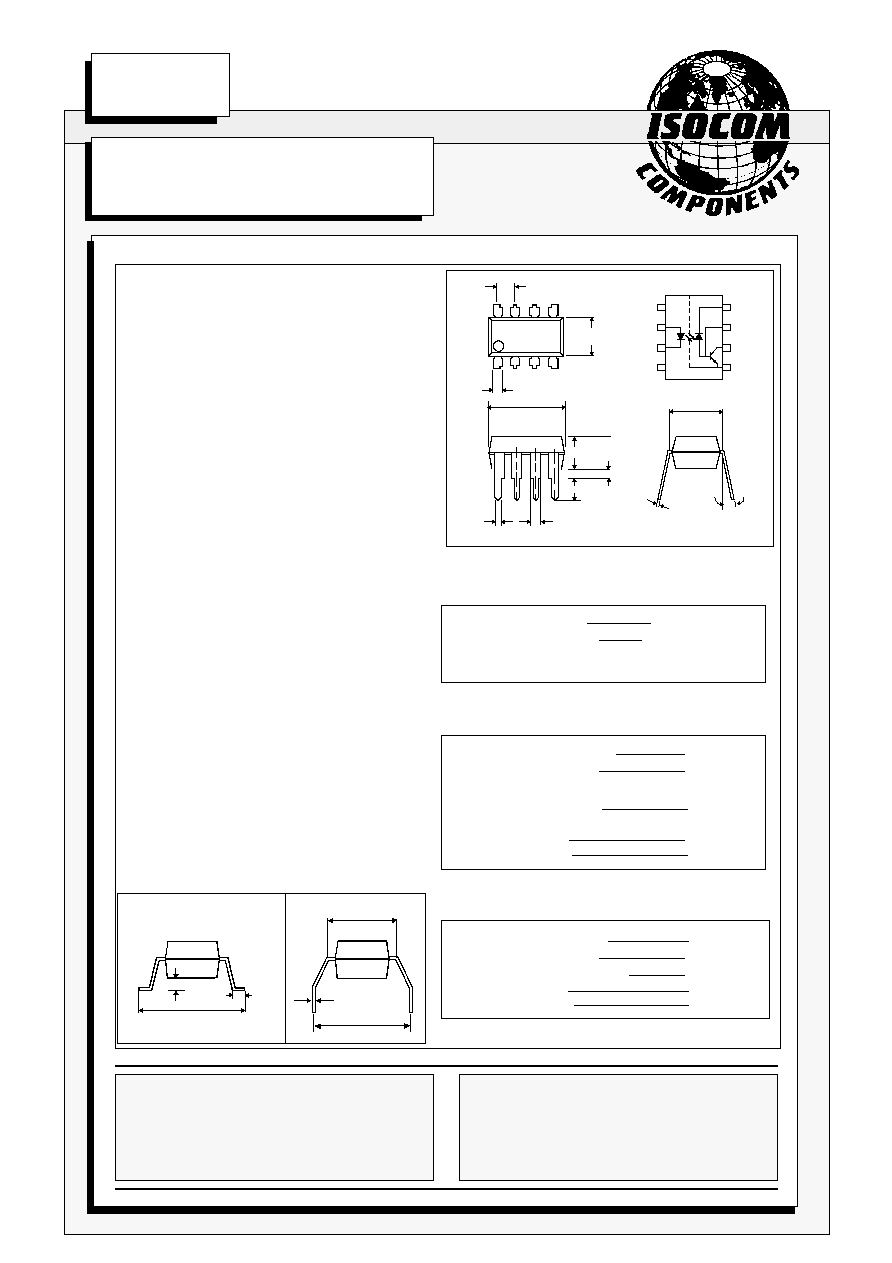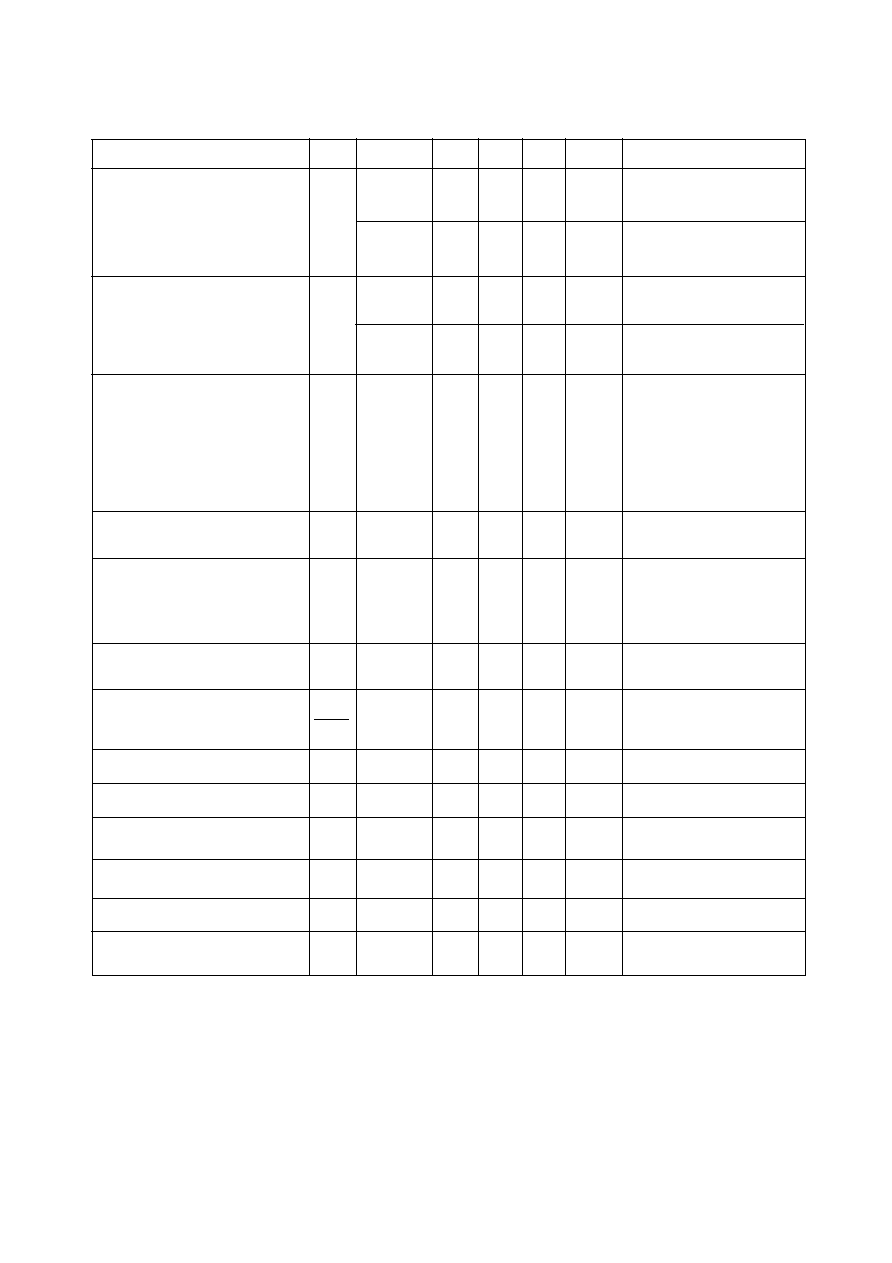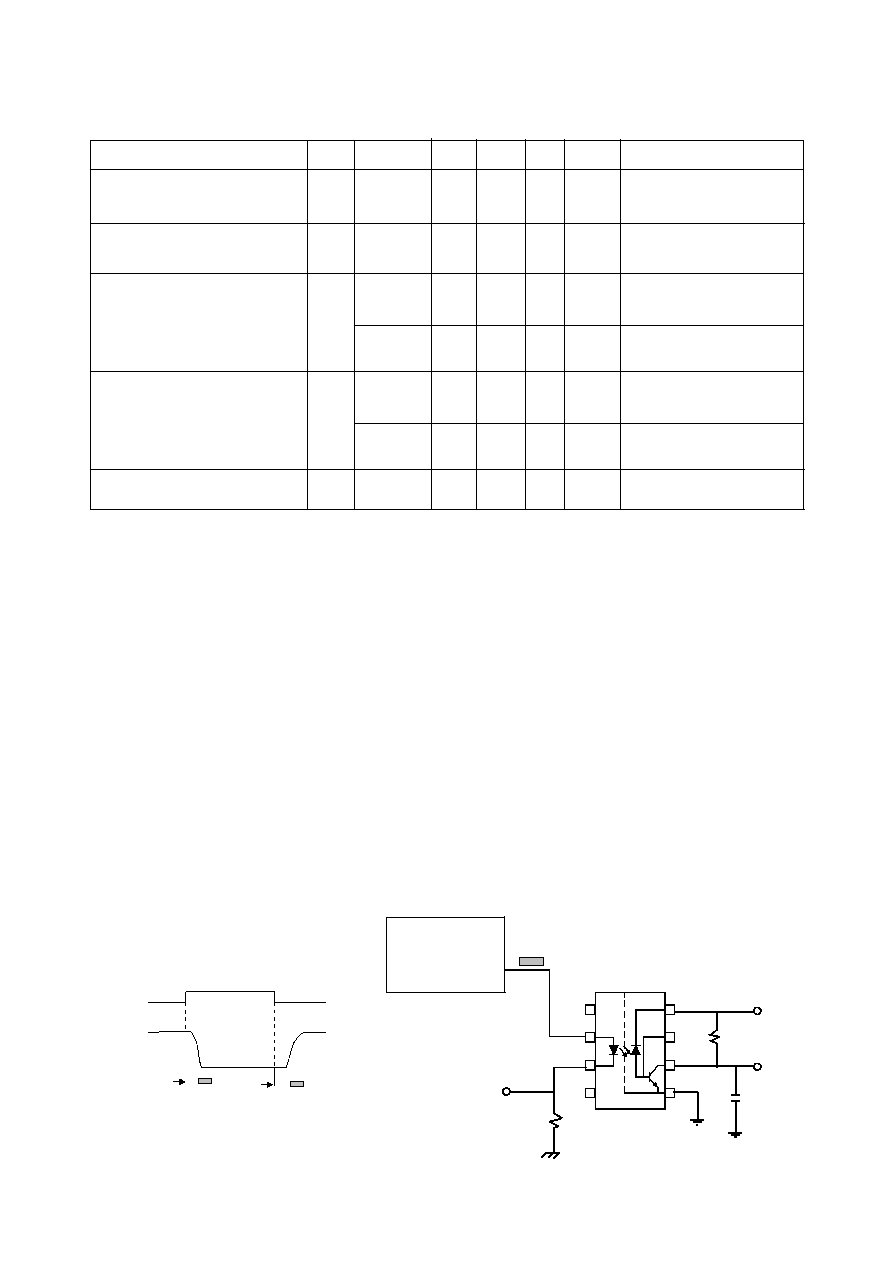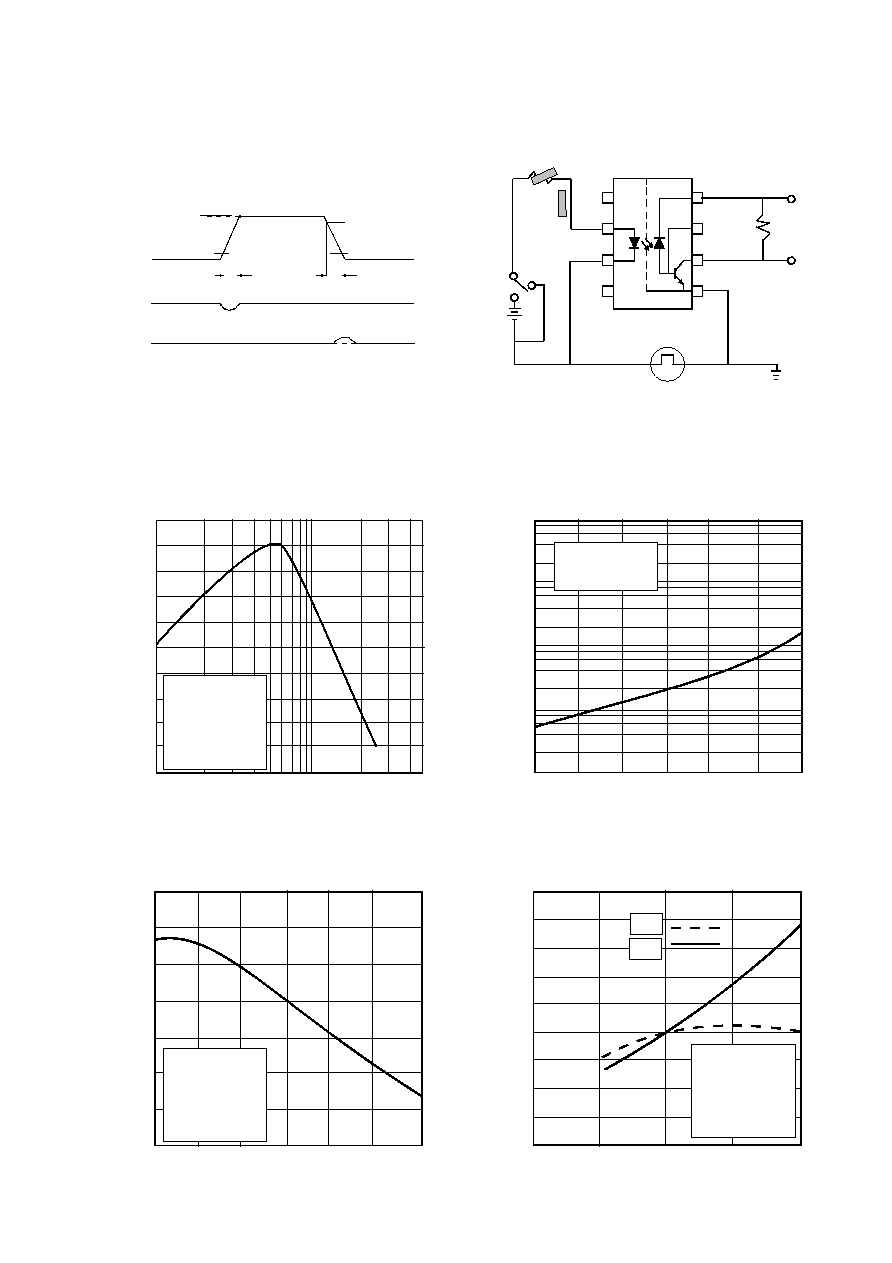Search datasheet (1.687.043 components) Search fieldPart namePart descriptionAbout site Manufacturers list ChipFindIC search engine AllXrefCross-reference database### Datasheet: 6N136 (Isocom Incorporated)

Download:PDFZIPISOCOM COMPONENTS LTD
Unit 25B, Park View Road West,
Park View Industrial Estate, Brenda Road
Hartlepool, Cleveland, TS25 1YD
Tel: (01429) 863609 Fax :(01429) 863581
27/1/00
DB91035-AAS/A1
6N135, 6N136
HIGH SPEED OPTICALLY
COUPLED ISOLATOR
PHOTOTRANSISTOR OUTPUT
ABSOLUTE MAXIMUM RATINGS
(25°C unless otherwise specified)
Storage Temperature
-55°C to + 125°C
Operating Temperature
-55°C to + 100°C
(1/16 inch (1.6mm) from case for 10 secs) 260°C
INPUT DIODE
Average Forward Current
25mA ( 1 )
Peak Forward Current
50mA ( 2 )
( 50% duty cycle, 1ms pulse width )
Peak Transient Current
1.0A
(equal to or less than 1
µ
s P.W., 300 pps)
Reverse Voltage
5V
Power Dissipation
45mW( 3 )
DETECTOR
Average Output Current
8mA
Peak Output Current
16mA
Supply and Output Voltage
-0.5 to +15V
Base Current
5mA
Power Dissipation
100mW(4 )
APPROVALS
l
UL recognised, File No. E91231
DESCRIPTION
These diode-transistor optocouplers use a light
emitting diode and an integrated photon detector to
provide 2500Volts
RMS
electrical isolation between
input and output. Seperate connection for the
photodiode bias and output transistor collector
improve the speed up to a hundred times that of a
conventional photo-transistor coupler by reducing
the base-collector capacitance.
FEATURES
l
High speed - 1 MBits/s
l
High Common Mode Transient
Immunity 1000V/
µ
s
l
TTL Compatible
l
2 MHz Bandwidth
l
Open Collector Output
l
2500V
RMS
Withstand Test Voltage,1 Min
l
6N136 has improved noise shield which
gives superior common mode rejection
l
Options :-
Surface mount - add SM after part no.
Tape&reel - add SMT&R after part no.
l
All electrical parameters 100% tested
l
Custom electrical selections available
APPLICATIONS
l
l
Pulse transformer replacement
l
Wide bandwidth analog coupling
l
Output interface to CMOS-LSTTL-TTL
0.3
0.5
Dimensions in mm
6.9
6.3
1.3
15°
Max
3.3
4.0
3.6
2.54
9.7
9.1
0.5
1.3
* 6N136 NOISE SHIELD
4
3
2
1
8
7
6
5
7.62
*
OPTION G
OPTION SM
10.16
10.2
9.5
0.3
1.2
0.6
1.4
0.9
7.62
SURFACE MOUNT
ISOCOM INC
1024 S. Greenville Ave, Suite 240,
Allen, TX 75002 USA
Tel: (214) 495-0755 Fax: (214) 495-0901
e-mail info@isocom.com
http://www.isocom.comDB91035-AAS/A1
27/1/00
ELECTRICAL CHARACTERISTICS ( T
A
= 0°C to 70°C Unless otherwise noted )
PARAMETER
SYM DEVICE
MIN TYP* MAX UNITS TEST CONDITION
6N135
7
18
%
I
F
= 16mA, V
O
= 0.4V
Current Transfer Ratio
CTR 6N136
19
24
%
V
CC
= 4.5V, T
A
= 25°C
(note 5)
6N135
5
19
%
I
F
= 16mA, V
O
= 0.5V
6N136
15
25
%
V
CC
= 4.5V
6N135
0.1
0.4
V
I
F
= 16mA, I
O
=1.1mA
Logic Low Output Voltage
V
OL
V
CC
= 4.5V, T
A
= 25°C
6N136
0.1
0.4
V
I
F
= 16mA, I
O
=2.4mA
V
CC
= 4.5V, T
A
= 25°C
3
500
nA
I
F
= 0mA, T
A
= 25°C
V
O
= V
CC
= 5.5V
Logic High Output Current
I
OH
0.01
1
µ
A
I
F
= 0mA, T
A
= 25°C
V
O
= V
CC
= 15V
50
µ
A
I
F
= 0mA
V
O
= V
CC
= 15V
Logic Low Supply Current
I
CCL
40
µ
A
I
F
= 16mA,V
O
= open
V
CC
= 15V
0.02
1
µ
A
I
F
= 0mA, V
O
= open
V
CC
= 15V, T
A
= 25°C
Logic High Supply Current
I
CCH
2
µ
A
I
F
= 0mA,V
O
= open
V
CC
= 15V
Input Forward Voltage
V
F
1.5
1.7
V
I
F
= 16mA, T
A
= 25°C
Temperature Coefficient
V
F
-1.6
mV/°C
I
F
= 16mA
of Forward Voltage
T
A
Input Reverse Voltage
V
R
5
V
I
R
= 10
µ
A,T
A
= 25°C
`
Input Capacitance
C
IN
60
pF
f
= 1MHz, V
F
= 0
Input-output Isolation Voltage
V
ISO
2500
5000
V
RMS
R.H.equal to or less than
50%, t = 1min. T
A
= 25°C
Resistance (Input to Output)
R
IO
10
12
V
IO
= 500V dc (note 6)
Capacitance (Input to Output)
C
IO
0.6
pF
f = 1MHz (note 6)
Transistor DC Current Gain
H
FE
150
V
O
= 5V, I
O
= 3mA
* All typicals at T
A
= 25°CSWITCHING SPECIFICATIONS AT T
A
= 25°C ( V
CC
= 5V, I
F
= 16mA Unless otherwise noted )
PARAMETER
SYM DEVICE
MIN TYP MAX UNITS TEST CONDITION
6N135
0.5
1.5
µ
s
R
L
= 4.1k
,
( note 9 )
Logic Low at Output ( fig 1 )
6N136
0.2
0.8
µ
s
R
L
= 1.9k
,
( note 8 )
Propagation Delay Time
t
PLH
6N135
0.5
1.5
µ
s
R
L
= 4.1k
,
( note 9 )
Logic High at Output ( fig 1)
6N136
0.2
0.8
µ
s
R
L
= 1.9k
,
( note 8 )
Common Mode Transient
6N135
1000
V/
µ
s
I
F
= 0mA, V
CM
= 10V
PP
Immunity at Logic High
R
L
= 4.1k
,
(note7,8,9)
Level Output ( fig 2 )
CM
H
6N136
1000
V/
µ
s
I
F
= 0mA, V
CM
= 10V
PP
R
L
= 1.9k
,
(note7,8,9)
Common Mode Transient
6N135
-1000
V/
µ
s
V
CM
= 10V
PP
Immunity at Logic Low
R
L
= 4.1k
,
(note7,8,9)
Level Output ( fig 2 )
CM
L
6N136
-1000
V/
µ
s
V
CM
= 10V
PP
R
L
= 1.9k
,
(note7,8,9)
Bandwidth
BW
2
MHz
R
L
= 100
,
(note 10 )
NOTES:-
1.
Derate linearly above 70
o
C free air temperature at a rate of 0.8 mA/°C.
2.
Derate linearly above 70
o
C free air temperature at a rate of 1.6 mA/°C.
3.
Derate linearly above 70
o
C free air temperature at a rate of 0.9 mW/°C.
4.
Derate linearly above 70
o
C free air temperature at a rate of 1.0 mW/°C.
5.
CURRENT TRANSFER RATIO is defined as the ratio of output collector current,I
O
, to the forward LED
input current, I
F
times 100%.
6.
Device considered a two-terminal device: pins 1,2,3, and 4 shorted together and pins 5,6,7 and 8 shorted
together.
7.
Common mode transient immunity in Logic High level is the maximum tolerable (positive) dVcm/dt on
the leading edge of the common mode pulse V
CM
to assure that the output will remain in a Logic High
state (i.e. V
O
> 2.0V). Common mode transient immunity in Logic Low level is the maximum tolerable
(negative) dVcm/dt on the trailing edge of the common mode pulse signal, V
CM
to assure that the output
will remain in Logic Low state (i.e. V
O
< 0.8V).
8.
The 1.9k
pull-up resistor.
9.
The 4.1k
pull-up resistor.
10.
The frequency at which the a.c. output voltage is 3dB below the low frequency asymptote.
Propagation Delay Time
t
PHL
FIG.1 SWITCHING TEST CIRCUIT
2
I
F
Monitor
0
I
F
V
O
1.5V
100
1.5V
5V
t
PHL
t
PLH
V
OL
R
L
V
O
C
L
= 15pF
8
7
6
5
I
F
1
4
3
10% Duty Cycle
1/f < 100
µ
s
5V
DB91035-AAS/A1
27/1/00
PULSE
GENERATOR
Z
O
= 50
t
r
= 5nsDB91035-AAS/A1
27/1/00
FIG. 2 TEST CIRCUIT FOR TRANSIENT IMMUNITY AND TYPICAL WAVEFORMS
1
3
4
+
-
A
PULSE GEN.
V
CM
0V
V
CM
I
F
V
FF
5V
5V
V
OL
V
O
V
O
SWITCH AT A: I
F
= 0mA
SWITCH AT B: I
F
= 16mA
10V
10%
90%
10%
90%
t
r
t
f
B
8
7
6
5
2
R
L
V
O
Logic high output current I
OH
(nA)
Logic High Output Current vs.
Ambient Temperature
1 2 5 10 20 50
0.9
1.0
1.1
1.2
1.3
Normalized current transfer ratio
Normalized Current Transfer
Ratio vs. Forward Current
Forward current I
F
(mA)
0.8
0.7
0.7
0.8
0.9
Normalized Current Transfer
Ratio vs. Ambient Temperature
Normalized current transfer ratio
Ambient temperature T
A
( °C )
-55 -25 0 25 50 75 100
-25 0 25 50 70
Ambient temperature T
A
( °C )
Normalized propagation delay
0.7
0.8
0.9
1.0
1.1
1.2
1.3
1.4
1.5
1.6
Normalized
to I
F
= 16mA
V
O
= 0.4V
V
CC
= 5V
T
A
= 25°C
1.0
1.1
1.2
1.3
-55 -25 0 25 50 75 100
Ambient temperature T
A
( °C )
I
F
= 0mA
V
O
= V
CC
= 5V
10
-1
10
0
10
1
10
-2
10
-3
t
PLH
t
PHL
1.4
1.5
Normalized Propagation Delay
vs. Ambient Temperature
Normalized
to I
F
= 16mA
V
O
= 0.4V
V
CC
= 5V
T
A
= 25°C
Normalized
to I
F
= 16mA
V
CC
= 5V
R
L
= 4.1k
T
A
= 25°C© 2020 • ICSheet• Contact form• Main page